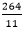# GCSE Maths – Averages

An average of a data set is a single value that typifies the data set in some way, and there are three distinct ways of defining this:

The Mode – the value that occurs most often in the data set.

The Median – the middle value when the data set is listed in ascending order.

The Mean – which is the sum of all the values in the data set divided by the number of values (i.e. in line with the everyday meaning of the word ‘average’).

As well as knowing the value of a ‘typical’ member of the data set it is useful to have a measure of the amount of variation in a data set. There are many measures of the amount of variation within a data set, the simplest of these is the range – the difference between the highest and lowest values in the data set.

Sample question

Give the mode, median, mean and range of the data set 20, 26, 21, 22, 23, 30, 26, 22, 23, 25, 26.

Mean = 24. The total of all the values is 264 and there are 11 values, so the mean